# Abstract wave equation

Consider the Cauchy problem for the wave equationwith the Dirichlet boundary conditions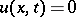or the Neumann boundary conditions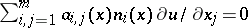,.

Here,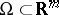is a bounded domain with smooth boundary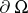,andare smooth real functions on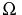such thatfor all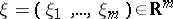, with some fixed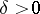;is the unit outward normal vector to. Also,,,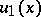are given functions. The functionis the unknown function.

One can state this problem in the abstract form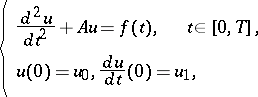(a1)

which is considered in the Hilbert space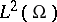. Here,is the self-adjoint operator ofdetermined from the symmetric sesquilinear form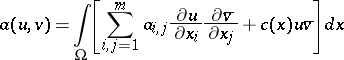(a2)

on the space, see [a1], where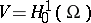(respectively) when the boundary conditions are Dirichlet (respectively, Neumann), by the relation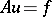if and only iffor all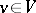. There are several ways to handle this abstract problem.

Letbe a Banach space. A strongly continuous function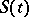of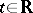with values in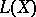is called a cosine function if it satisfies,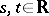, and. Its infinitesimal generatoris defined by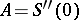, with. The theory of cosine functions, which is very similar to the theory of semi-groups, was originated by S. Kurera [a2] and was developed by H.O. Fattorini [a3] and others.

A necessary and sufficient condition for a closed linear operatorto be the generator of a cosine family is known. The operator determined by (a2) is easily shown to generate a cosine function which provides a fundamental solution for (a1).

Suppose one setsin (a1). Then one obtains the equivalent problemwhich is considered in the product space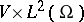. Since the equation is of first order, one can apply semi-group theory (see [a4], [a5]). Indeed, the operatorwith its domainis the negative generator of a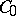semi-group. The theory of semi-groups of abstract evolution equations provides the existence of a unique solution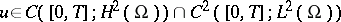of (a1) forand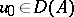,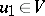.

This method is also available for a non-autonomous equation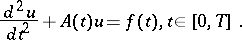(a3)

In the case of Neumann boundary conditions, the difficulty arises that the domain ofmay change with. One way to avoid this is to introduce the extension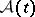of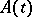defined byfor all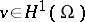. Sinceis a bounded operator from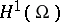into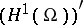, the operator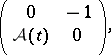acting in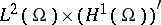, has constant domain.

Another way is to reduce (a3) toby setting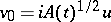,, under the assumption that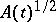is strongly differentiable with values in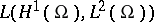. Obviously, the linear operator of the coefficient has constant domain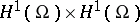. Differentiability of the square rootwas studied in [a6], [a7].

In order to consider in (a1) the case when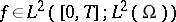, one has to use the Lions–Magenes variational formulation. In this, one is concerned with the solution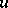of the problem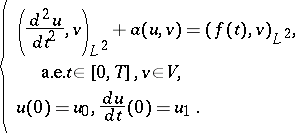The existence of a unique solutionhas been proved if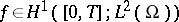and,; see [a8], Chap. 5.

This method is also available for a non-autonomous equation (a3).

The variational method enables one to take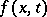from a wide class, an advantage that is very useful in, e.g., the study of optimal control problems. On the other hand, the semi-group method provides regular solutions, which is often important in applications to non-linear problems. Using these approaches, many papers have been devoted to non-linear wave equations.